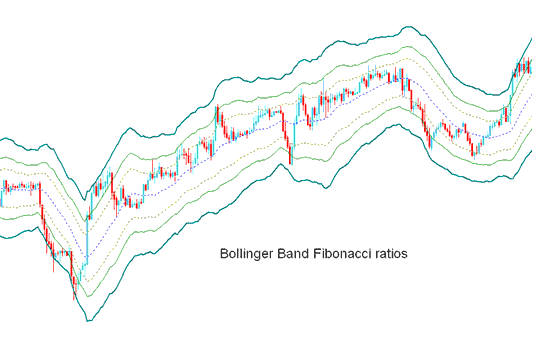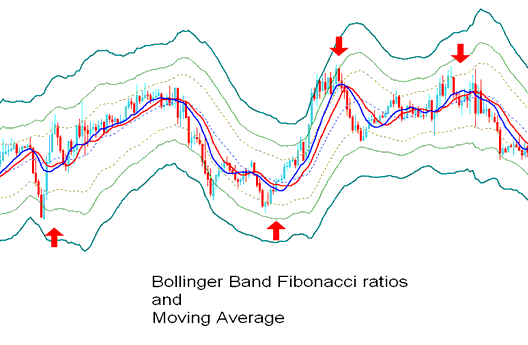# Fibonacci Ratios Stock Indices Technical Analysis and Stock Indices Signals

Derived from the original Bollinger bands.

The Bollinger Fibonacci ratios is a volatility based indicators but it does not use the standard deviation to calculate the width of the bands instead it uses a smoothed ATR that are multiplied with Fibonacci ratios of 1.618, 2.618, and 4.236.

The smoothed lines that are multiplied with Fibonacci ratios are then added or subtracted from the moving average.

This forms 3 upper Fibonacci bands and 3 lower Fibonacci bands

The middle band forms the basis of the trend.## Stock Indices Technical Analysis and Generating Stock Indices Signals

This indicator used to determine point of support and resistance for a stock indices.

The lines below represent support points while those above are resistance levels.

The outermost bands provide the strongest resistance/support.

The inner most bands provide least support/resistance.

The innermost band represents Fibonacci 38.2 % retracement level

The second band represents Fibonacci 50 % retracement level

The outermost band represents Fibonacci 61.8 % retracement level

This technical indicator is used to determine points where stock index price might reverse. (Indices Price Pullback Levels)

When stock index price hits one of the lines and reverses then an entry or exit signal is generated.

However, it is always good to combine the signal with other confirmation indicators such as the moving average to confirm the signal as shown below.Technical Analysis